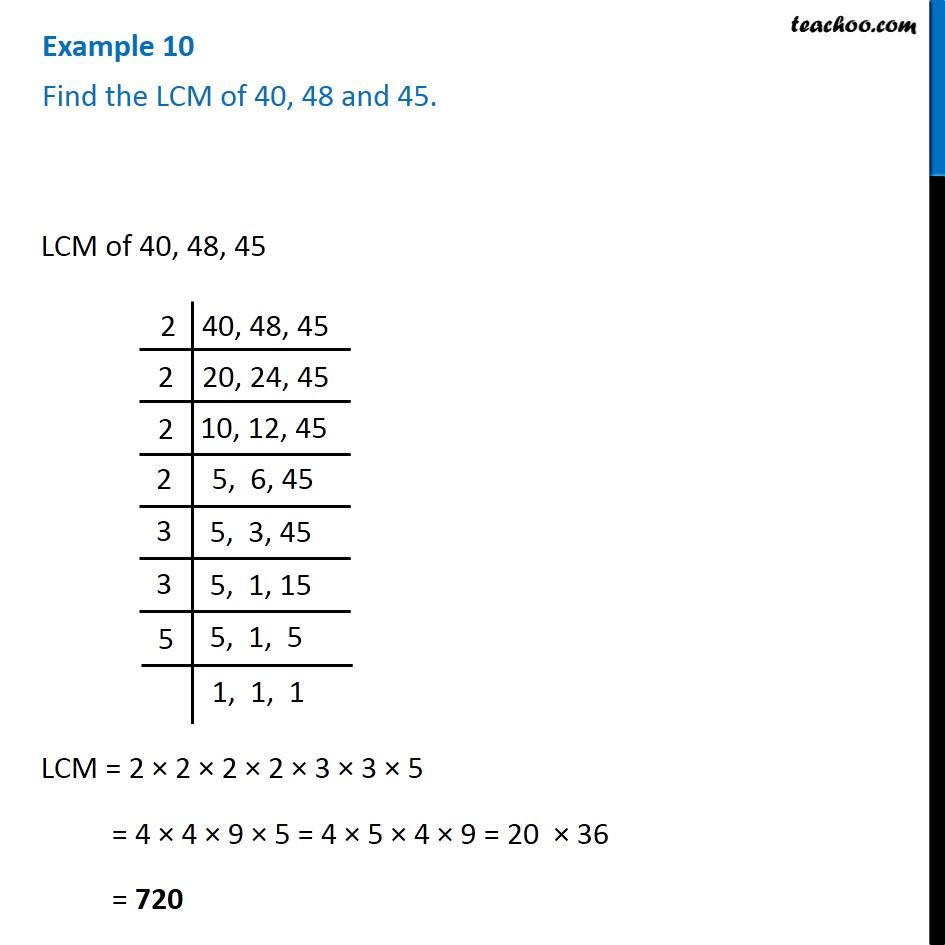1. Chapter 3 Class 6 Playing with Numbers
2. Serial order wise
3. Examples

Transcript

Example 10 Find the LCM of 40, 48 and 45. LCM of 40, 48, 45 2|40, 48, 45 2|20, 24, 45 2|10, 12, 45 2| 5, 6, 45 3| 5, 3, 45 3| 5, 1, 15 5| 5, 1, 5 5| 1, 1, 1 LCM = 2 × 2 × 2 × 2 × 3 × 3 × 5 = 4 × 4 × 9 × 5 = 4 × 5 × 4 × 9 = 20 × 36 = 720

Examples

Chapter 3 Class 6 Playing with Numbers
Serial order wise